## Filters

Sort by :
Clear All
Q

Find the difference between and .

the difference between and  is given by

Sohan was putting covers on his note books. He put one fourth of the covers on Monday. He put another one fourth on              Tuesday and the remaining on Wednesday. What fraction of the covers did he put on Wednesday?

He put covers on Monday= He put the cover on Tuesday = and the remaining on Wednesday. Thus, the fraction of the covers he put on Wednesday =

Mother asked Neelu and her brother to pick stones from the wheat. Neelu picked one-fourth of the total stones in it and her brother also picked up one-fourth of the stones. What fraction of the stones did both pick up together?

. Neelu picked stones       brother picked up the stones The total fraction of the stones they both pick up together  of total stones.

My mother divided an apple into equal parts. She gave me two parts and my brother one part. How much apple did she give to both of us together?

mother gave to me  part mother gave to my brother   part She gave both off us    part

Arrange the following in ascending and descending order :

(c) Write 3 more similar examples and arrange them in ascending and descending order.

the following in ascending and descending order are: (i)  and  (ii)  and  (iii)  and

Q. 1.     Arrange the following in ascending and descending order :

(b)

the following in ascending and descending order are  :             (b)

Arrange the following in ascending and descending order :

(a)

(a)                    and

A triangular prism looks like the shape of a Kaleidoscope. It has triangles as its bases.

Faces : _______

Edges : _______

Corners : _______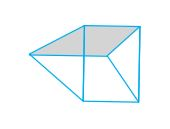Faces =   (Two triangular faces and three square faces) Edges = 9     Corners =

A square pyramid has a square as its base.

Faces : _______

Edges : _______

Corners : _______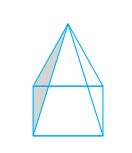In a square pyramid, the number of Faces =           (Four triangular faces and one square face) Edges =           (Four edges of the square base and other four joining at the top)    Corners =

A triangular pyramid has a triangle as its base. It is also known as a tetrahedron.

Faces : _______

Edges : _______

Corners : _______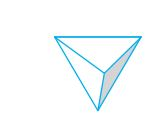The number of Faces =           (All triangular faces) Edges =               Corners =

A cube is a cuboid whose edges are all of equal length.

It has ______ faces.

Each face has ______ edges.

Each face has ______ vertices.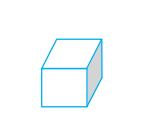It has  faces.        (Three pairs of parallel square faces)   Each face has  edges.          Each face has  vertices

Using four unequal sticks, as you did in the above activity, see if you can form a quadrilateral such that

(a) all the four angles are acute.

(b) one of the angles is obtuse.

(c) one of the angles is right angled.

(d) two of the angles are obtuse.

(e) two of the angles are right angled.

(f) the diagonals are perpendicular to one another

(a) all the four angles are acute.             (b) one of the angles is obtuse.             (c) one of the angles is right angled.             (d) two of the angles are obtuse.               (e) two of the angles are right angled.               (f) the diagonals are perpendicular to one another

Place a pair of unequal sticks such that they have their end points joined at one end. Now place another such pair meeting the             free ends of the first pair. What is the figure enclosed? It is a quadrilateral, like the one you see here. The sides of the                      quadrilateral are AB, BC , ___, ___. There are 4 angles for this quadrilateral. They are given by ∠BAD, ∠ADC, ∠DCB and _____.            BD is one diagonal. What is the other? Measure the length of the sides and the diagonals. Measure all the angles also.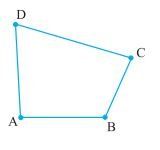The sides of the quadrilateral are AB, BC, CD, DA The angles are given by The other diagonal is AD.

Try to draw rough sketches of

(a) a scalene acute angled triangle.

(b) an obtuse angled isosceles triangle.

(a) a scalene acute angled triangle. :  Scalene : All side of different length Acute angled : All angles less than    (b) an obtuse angled isosceles triangle Isosceles traingle: Only two sides are of equal length Obtuse angled : At least one angle greater than

What does the angle made by the hour hand of the clock look like when it moves from 5 to 7. Is the angle moved more than 1                   right angle?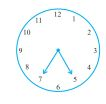No, the angle is not more than than 1 right angle.  For each hour, angle made =  Therefore, when the hour hand moves from 5 to 7, the angle made =

The hour hand of a clock moves from 12 to 5. Is the revolution of the hour hand more than 1 right angle?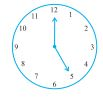Yes, the revolution of the hour hand is more than 1 right angle. For each hour, angle made =  Therefore, when the hour hand moves from 12 to 5, the angle made =

Draw five other situations of one-fourth, half and three-fourth revolution on a clock.

(a) One fourth revolution: From (b) Half revolution: From (c) Three fourth revolution: From (d) Three fourth revolution: From (e) Half fourth revolution: From

What is the angle name for one-fourth revolution?

One-fourth revolution =  The angle name for one-fourth revolution is "Right Angle"

What is the angle name for half a revolution?

Half a revolution =  The angle name for half a revolution is "Straight Angle".

Read the table and fill in the boxes.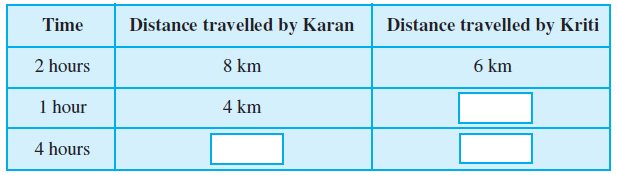distance travelled by kriti in 1 hour is 3km

distance travelled by karan in 4 hours is 16km

distance travelled by kriti in 4 hours is 12km

Exams
Articles
Questions